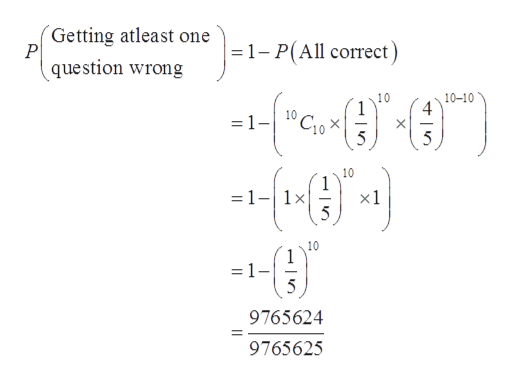# On a 10 question multiple-choice test, where each question has 5 answers, what would be the probability of getting at least one question wrong?Give your answer as a fraction

Question
17 views

On a 10 question multiple-choice test, where each question has 5 answers, what would be the probability of getting at least one question wrong?

check_circle

Step 1

The provided information are:

Probability of correct answer (p) = 1/5

Probability of incorrect answer (q) = 4/5

Number of questions (n) = 10

Step 2

The probability of getting at least one q...help_outlineImage TranscriptioncloseGetting atleast one 1- P(All correct question wrong 10 1 10-10 4 =1- 5 10 1 x1 1x 10 1 = 1 9765624 9765625 fullscreen

### Want to see the full answer?

See Solution

#### Want to see this answer and more?

Solutions are written by subject experts who are available 24/7. Questions are typically answered within 1 hour.*

See Solution
*Response times may vary by subject and question.
Tagged in

### Other